POINT OF INTEREST 6
DIFFERENCE , DOUBLE, AND HALF ANGLE IDENTITIESProof of the Difference Identity for CosineWe will use the following picture for this proof:Since the angles between the segment OA and OC and the segment OB and OD both measure (u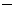v), the arcs AC and BD must have the same length. This implies that the line segments connecting points A and C and points B and D are also equal in length.  That is,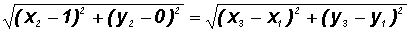Raising both sides to the second power and combining like terms, we getNotice that the parentheses contain circles. The value of the three quantities within the parentheses is 1 since,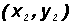, and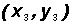are points on a unit circle.

Therefore,Finally, letting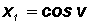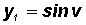we get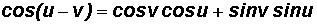, which is usually presented as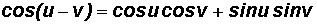Proof of the Sum and Difference Identities for Sine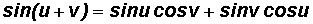and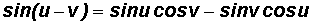Given the Difference Identity for Cosine, it is easy to show that. We will use this fact to show a proof of the Sum and Difference Identities for Sine.

If we let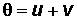, then we can prove a Sum Identity for Sine!If we let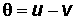, then we can prove a Difference Identity.Incidentally, the Sum Identity for Cosine can be established using.

Proof of the Double Angle Identities for

Sine

To proof the first identity, let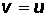in the Sum Identity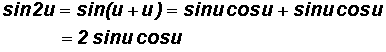Cosine

To proof the second identity, letin the Sum Identity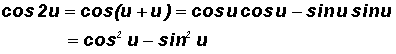Proof of the Half Angle Identities for

Sine

Here we use the Double Angle IdentityFinally, let, thenCosine

Here we use the Double Angle Identity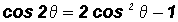Finally, let, then